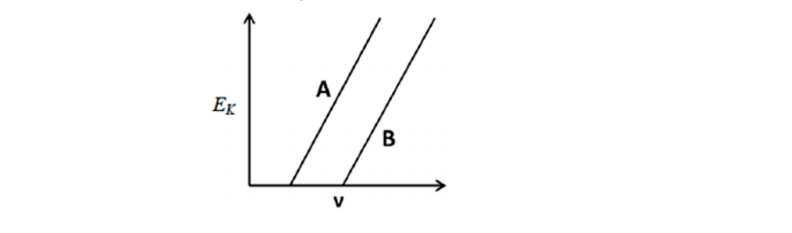# Problem: The figure below shows Ek plotted as a function of v for photoelectric emission from two metals, A and B. Which of the following statements for the two metals is correct? A. Both metals will produce the approximately equal currents under identical conditions. B. The threshold frequencies suggest that metal A is an alkali metal. C. The threshold frequency is the same for both metals. D. The threshold frequency of A is greater than that of B. E. The threshold frequency of A is less than that of B.

###### Problem Details

The figure below shows Ek plotted as a function of v for photoelectric emission from two metals, A and B. Which of the following statements for the two metals is correct?

A. Both metals will produce the approximately equal currents under identical conditions.

B. The threshold frequencies suggest that metal A is an alkali metal.

C. The threshold frequency is the same for both metals.

D. The threshold frequency of A is greater than that of B.

E. The threshold frequency of A is less than that of B.